JMSLTM Numerical Library 7.2.0
com.imsl.stat

## Class PartialCovariances

• All Implemented Interfaces:
Serializable

```public class PartialCovariances
extends Object
implements Serializable```

Class `PartialCovariances` computes the partial covariances or partial correlations from an input covariance or correlation matrix. If the "independent" variables (the linear "effect" of the independent variables is removed in computing the partial covariances/correlations) are linearly related to one another, `PartialCovariances` detects the linearity and eliminates one or more of the independent variables from the list of independent variables. The number of variables eliminated, if any, can be determined from `getPartialDegreesOfFreedom`.

Given a covariance or correlation matrix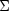partitioned as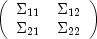class `PartialCovariances` computes the partial covariances (of the standardized variables ifis a correlation matrix) as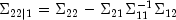A positive semidefinite solver is used to compute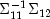.

If partial correlations are desired, these are computed as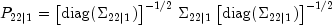where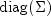denotes the matrix containing the diagonal of its argument along its diagonal with zeros off the diagonal. If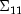is singular, then as many variables as required are deleted from(and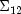) in order to eliminate the linear dependencies. The computations then proceed as above.

The p-value for a partial covariance tests the null hypothesis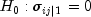, where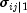is the (i, j) element in matrix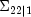. The p-value for a partial correlation tests the null hypothesis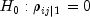, where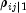is the (i, j) element in matrix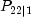. The p-values are returned by `getPValues`. If the degrees of freedom for `sigma`, `df`, is not known, the resulting p-values may be useful for comparison, but they should not by used as an approximation to the actual probabilities.

Example1, Example2, Serialized Form
• ### Nested Class Summary

Nested Classes
Modifier and Type Class and Description
`static class ` `PartialCovariances.InvalidMatrixException`
Exception thrown if a computed correlation is greater than one for some pair of variables.
`static class ` `PartialCovariances.InvalidPartialCorrelationException`
Exception thrown if a computed partial correlation is greater than one for some pair of variables.
• ### Constructor Summary

Constructors
Constructor and Description
```PartialCovariances(int[] xIndices, double[][] sigma, int df)```
Creates a `PartialCovariances` object from a covariance or correleation matrix with a mix of dependent and independent variables.
```PartialCovariances(int nIndependent, double[][] sigma, int df)```
Creates a `PartialCovariances` object from a covariance or correleation matrix with a the independent variables in the initial columns and the dependent variables in the final columns.
• ### Method Summary

Methods
Modifier and Type Method and Description
`double[][]` `getPartialCorrelationMatrix()`
Returns the partial correlation matrix.
`double[][]` `getPartialCovarianceMatrix()`
Returns the partial covariance matrix.
`int` `getPartialDegreesOfFreedom()`
Returns the degrees of freedom in the test that the partial correlation (covariance) is zero.
`double[][]` `getPValues()`
Calculates the p-values for testing the null hypothesis that the associated partial covariance/correlation is zero.
• ### Methods inherited from class java.lang.Object

`clone, equals, finalize, getClass, hashCode, notify, notifyAll, toString, wait, wait, wait`
• ### Constructor Detail

• #### PartialCovariances

```public PartialCovariances(int[] xIndices,
double[][] sigma,
int df)
throws PartialCovariances.InvalidMatrixException,
PartialCovariances.InvalidPartialCorrelationException```
Creates a `PartialCovariances` object from a covariance or correleation matrix with a mix of dependent and independent variables.
Parameters:
`xIndices` - is an array containing values indicating the status of the variable. If the i-th entry is 0 then the i-th column of the matrix contains a dependent variable. If the i-th entry is positive then the i-th column of the matrix contains an independent variable. If the i-th entry is negative then the i-th column of the matrix is not used in the analysis.
`sigma` - is a correlation or covariance matrix. The number of rows and columns in `sigma` must equal the length of the array `xIndices`. The matrix must always be symmetric, positive semidefinite.
`df` - is an `int` indicating the number of degrees of freedom associated with the input matrix. If the number of degrees of freedom in the matrix varies from element to element, then a conservative choice for `df` is the minimum degrees of freedom for all elements in the matrix. The value of `df` must be at least one.
Throws:
`PartialCovariances.InvalidMatrixException` - is thrown if a computed correlation is greater than one for some pair of variables.
`PartialCovariances.InvalidPartialCorrelationException` - is thrown if a computed partial correlation is greater than one for some pair of variables. The input matrix to the constructor was not positive semidefinite.
• #### PartialCovariances

```public PartialCovariances(int nIndependent,
double[][] sigma,
int df)
throws PartialCovariances.InvalidMatrixException,
PartialCovariances.InvalidPartialCorrelationException```
Creates a `PartialCovariances` object from a covariance or correleation matrix with a the independent variables in the initial columns and the dependent variables in the final columns.
Parameters:
`nIndependent` - is the number of "independent" variables to be used in the partial covariances/correlations. The partial covariances/correlations are the covariances/correlations between the dependent variables after removing the linear effect of the independent variables.
`sigma` - is a correlation or covariance matrix. The rows/columns must be ordered such that the first `nIndependent` rows/columns contain the independent variables, followed by the row/columns containing the dependent variables. The matrix must always be symmetric, positive semidefinite.
`df` - is an `int` indicating the number of degrees of freedom associated with the input matrix. If the number of degrees of freedom in the matrix varies from element to element, then a conservative choice for `df` is the minimum degrees of freedom for all elements in the matrix. The value of `df` must be at least one.
Throws:
`PartialCovariances.InvalidMatrixException` - is thrown if a computed correlation is greater than one for some pair of variables.
`PartialCovariances.InvalidPartialCorrelationException` - is thrown if a computed partial correlation is greater than one for some pair of variables. The input matrix to the constructor was not positive semidefinite.
• ### Method Detail

• #### getPartialCorrelationMatrix

`public double[][] getPartialCorrelationMatrix()`
Returns the partial correlation matrix. This is valid only if the input to the constructor was a correlation matrix.
Returns:
The partial correlation matrix.
• #### getPartialCovarianceMatrix

`public double[][] getPartialCovarianceMatrix()`
Returns the partial covariance matrix. This is valid only if the input to the constructor was a covariance matrix.
Returns:
The partial covariance matrix.
• #### getPartialDegreesOfFreedom

`public int getPartialDegreesOfFreedom()`
Returns the degrees of freedom in the test that the partial correlation (covariance) is zero. This will usually be `df` minus the rank of the the independent variables. number of independent variables, but will be greater than this value if the independent variables are computationally linearly related.
Returns:
An `int` scalar value representing the degrees of freedom. If this value is not greater than one then there are not enough degrees of freedom for hypothesis testing. A warning is also issued in this case.
• #### getPValues

`public double[][] getPValues()`
Calculates the p-values for testing the null hypothesis that the associated partial covariance/correlation is zero. It is assumed that the observations from which `sigma` was computed follows a multivariate normal distribution and that each element in `sigma` has `df` degrees of freedom.
Returns:
A square array of type `double` containing the p-values. The order of the matrix equals the number of dependent variables.

If the partial degrees of freedom is not greater than one then there are not enough degrees of freedom for hypothesis testing. The returned matrix will be set to all NaN values in this case. A warning is also issued in this case.

JMSLTM Numerical Library 7.2.0